# Formulating the CPMPERT Network as a Linear Programming Model[Page 357 ( continued )]

### Formulating the CPM/PERT Network as a Linear Programming Model

First we will look at the linear programming formulation of the general CPM/PERT network model and then at the formulation of the project crashing network.

We will formulate the linear programming model of a CPM/PERT network, using the AOA convention. As the first step in formulating the linear programming model, we will define the decision variables . In our discussion of CPM/PERT networks using the AOA convention, we designated an activity by its start and ending node numbers . Thus, an activity starting at node 1 and ending at node 2 was referred to as activity 1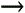2. We will use a similar designation to define the decision variables of our linear programming model.

We will use a different scheduling convention. Instead of determining the earliest activity start time for each activity, we will use the earliest event time at each node. This is the earliest time that a node ( i or j ) can be realized. In other words, it is the earliest time that the event the node represents, either the completion of all the activities leading into it or the start of all activities leaving it, can occur. Thus, for an activity ij , the earliest event time of node i will be x i , and the earliest event time of node j will be x j .

The objective function for the linear programming model is to minimize project duration.

The objective of the project network is to determine the earliest time the project can be completed (i.e., the critical path time). We have already determined from our discussion of CPM/PERT network analysis that the earliest event time of the last node in the network equals the critical path time. If we let x i equal the earliest event times of the nodes in the network, then the objective function can be expressed as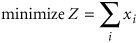[Page 358]

Because the value of Z is the sum of all the earliest event times, it has no real meaning; however, it will ensure the earliest event time at each node.

Next , we must develop the model constraints. We will define the time for activity ij as t ij (as we did earlier in this chapter). From our previous discussion of CPM/PERT network analysis, we know that the difference between the earliest event time at node j and the earliest event time at node i must be at least as great as the activity time t ij . A set of constraints that expresses this condition is defined as

x j x i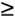t ij

The general linear programming model of formulation of a CPM/PERT network can be summarized as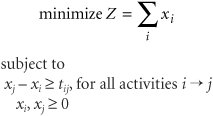where

x i = earliest event time of node i

x j = earliest event time of node j

t ij = time of activity ij

The solution of this linear programming model will indicate the earliest event time of each node in the network and the project duration.

As an example of the linear programming model formulation and solution of a project network, we will use the house-building network that we used to demonstrate project crashing. This network, with activity times in weeks and earliest event times, is shown in Figure 8.24.

##### Figure 8.24. CPM/PERT network for the house-building project, with earliest event times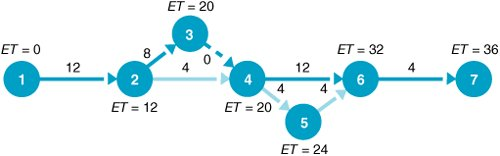The linear programming model for the network in Figure 8.24 is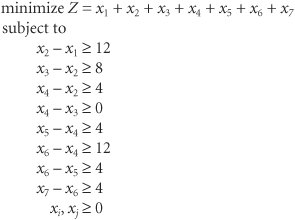Notice in this model that there is a constraint for each activity in the network.

[Page 359]

#### Solution of the CPM/PERT Linear Programming Model with Excel

The linear programming model of the CPM/PERT network in the preceding section enables us to use Excel to schedule the project. Exhibit 8.17 shows an Excel spreadsheet set up to determine the earliest event times for each nodethat is, the x i and x j values in our linear programming model for our house-building example. The earliest start times are in cells B6:B12 . Cells F6:F13 contain the model constraints. For example, cell F6 contains the constraint formula =B7B6 , and cell F7 contains the formula =B8B7 . These constraint formulas will be setto the activity times in column G when we access Solver. (Also, because activity 34 is a dummy , a constraint for F9=0 must be added to Solver.)

##### Exhibit 8.17.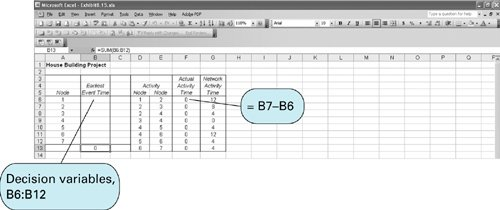Solver is accessed from the "Tools" menu located at the top of the spreadsheet. The Solver Parameters window with the model data is shown in Exhibit 8.18. Notice that the objective is to minimize the project duration in cell B13, which actually contains the earliest event time for node 7.

##### Exhibit 8.18.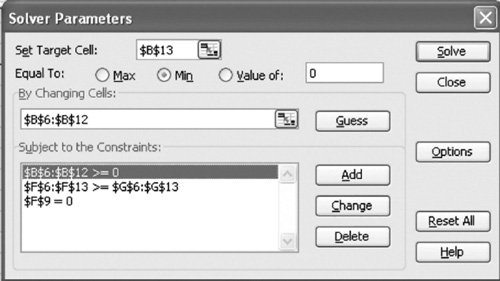The solution is shown in Exhibit 8.19. Notice that the earliest time at each node is given in cells B6:B12 , and the total project duration is 36 weeks. However, this output does not indicate the critical path. The critical path can be determined by accessing the sensitivity report for this problem. Recall that when you click on "Solve" from Solver, a screen comes up, indicating that Solver has reached a solution. This screen also provides the opportunity to generate several different kinds of reports , including an answer report and a sensitivity report. When we click on "Sensitivity" under the report options, the information shown in Exhibit 8.20 is provided.

[Page 360]
##### Exhibit 8.19.##### Exhibit 8.20.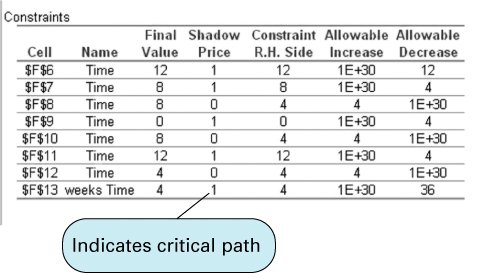The information in which we are interested is the shadow price for each of the activity constraints. (Remember that to get the shadow prices on the sensitivity report, you must click on "Options" from Solver and then select "Assume Linear Model." This is covered in Chapter 3, on solving linear programming problems with Excel.) The shadow price for each activity will be either 1 or 0. A positive shadow price of 1 for an activity means that you can reduce the overall project duration by an amount with a corresponding decrease by the same amount in the activity duration. Alternatively, a shadow price of 0 means that the project duration will not change, even if you change the activity duration by some amount. This means that those activities with a shadow price of 1 are on the critical path. Cells F6, F7, F9, F11, and F13 have shadow prices of 1, and referring back to Exhibit 8.19, we see that these cells correspond to activities 12, 23, 34, 46, and 67, which are the activities on the critical path.

#### Project Crashing with Linear Programming

The linear programming model required to perform project crashing analysis differs from the linear programming model formulated for the general CPM/PERT network in the previous section. The linear programming model for project crashing is somewhat longer and more complex.

The objective of the project crashing model is to minimize the cost of crashing .

The objective for our general linear programming model was to minimize project duration; the objective of project crashing is to minimize the cost of crashing, given the limits on how much individual activities can be crashed. As a result, the general linear programming model formulation must be expanded to include crash times and cost. We will continue to define the earliest event times for activity ij as x i and x j . In addition, we will define the amount of time each activity ij is crashed as y ij . Thus, the decision variables are defined as

[Page 361]

x i = earliest event time of node i

x j = earliest event time of node j

y ij = amount of time by which activity ij is crashed (i.e., reduced)

The objective of project crashing is to reduce the project duration at the minimum possible crash cost. For our house-building network, the objective function is written as

minimize Z = \$400 y 12 + 500 y 23 + 3,000 y 24 + 200 y 45 + 7,000 y 46 + 200 y 56 + 7,000 y 67

The objective function coefficients are the activity crash costs per week from Table 8.4; the variables y ij indicate the number of weeks each activity will be reduced. For example, if activity 12 is crashed by 2 weeks, then y 12 = 2, and a cost of \$800 is incurred.

The model constraints must specify the limits on the amount of time each activity can be crashed. Using the allowable crash times for each activity from Table 8.4 enables us to develop the following set of constraints:

 y 12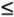5 y 233 y 241 y 34y 453 y 463 y 563 y 671

For example, the first constraint, y 125, specifies that the amount of time by which activity 12 is reduced cannot exceed 5 weeks.

The next group of constraints must mathematically represent the relationship between earliest event times for each activity in the network, as the constraint x j x it ij did in our original linear programming model. However, we must now reflect the fact that activity times can be crashed by an amount y ij . Recall the formulation of the activity 12 constraint for the general linear programming model formulation in the previous section:

x 2 x 112

This constraint can also be written as

x 1 + 12x 2

This latter constraint indicates that the earliest event time at node 1 ( x 1 ), plus the normal activity time (12 weeks) cannot exceed the earliest event time at node 2 ( x 2 ). To reflect the fact that this activity can be crashed, it is necessary only to subtract the amount by which it can be crashed from the left-hand side of the preceding constraint: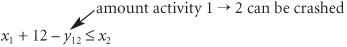This revised constraint now indicates that the earliest event time at node 2 ( x 2 ) is determined not only by the earliest event time at node 1 plus the activity time but also by the amount the activity is crashed. Each activity in the network must have a similar constraint, as follows :

[Page 362]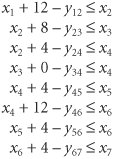Finally, we must indicate the project duration we are seeking (i.e., the crashed project time). Because the housing contractor wants to crash the project from the 36-week normal critical path time to 30 weeks, our final model constraint specifies that the earliest event time at node 7 should not exceed 30 weeks:

x 730

The complete linear programming model formulation is summarized as follows: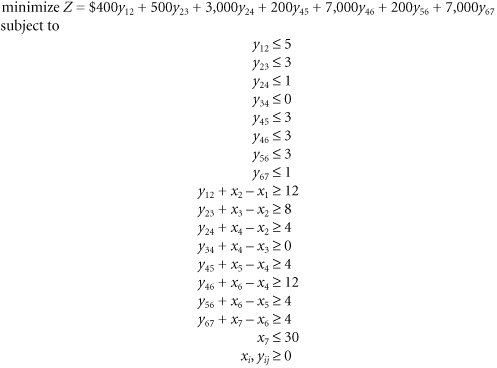#### Project Crashing with Excel

Because we have been able to develop a linear programming model for project crashing, we can also solve this model by using Excel. Exhibit 8.21 shows a modified version of the Excel spreadsheet we developed earlier, in Exhibit 8.16, to determine the earliest event times for our CPM/PERT network for the house-building project. We have added columns H, I, and J for the activity crash costs, the activity crash times, and the actual activity crash times. Cells J6:J13 correspond to the y ij variables in the linear programming model. The constraint formulas for each activity are included in cells F6:F13 . For example, cell F6 contains the formula =J6+B7B6 , and cell F7 contains the formula =J7+B8B7 . These constraints and the others in column F must be setto the activity times in column G. The objective function formula in cell B16 is shown on the formula bar at the top of the spreadsheet. The crashing goal of 30 weeks is included in cell B15.

[Page 363]
##### Exhibit 8.21.The problem in Exhibit 8.21 is solved by using Solver, as shown in Exhibit 8.22. Notice that there are two sets of variables in cells B6:B12 and J6:J13 . The project crashing solution is shown in Exhibit 8.23.

##### Exhibit 8.22.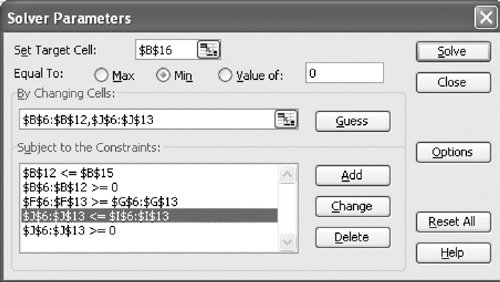##### Exhibit 8.23.Introduction to Management Science (10th Edition)
ISBN: 0136064361
EAN: 2147483647
Year: 2006
Pages: 358

Similar book on Amazon

flylib.com © 2008-2017.
If you may any questions please contact us: flylib@qtcs.net